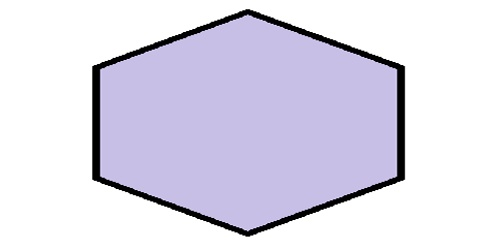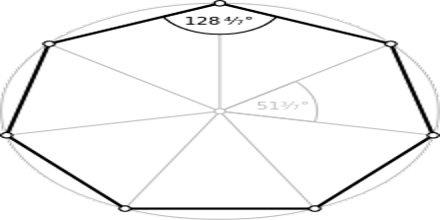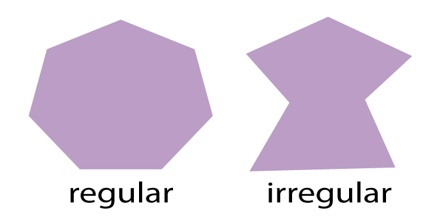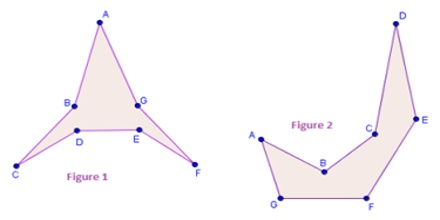Mathematic

# Heptagon Polygon: Definition with TypesHeptagon is a type of polygon that is composed of 7 lines bounded together. It is a two-dimensional figure. Therefore, a heptagon has seven sides as well as seven angles. The angles are always found at the vertices. It is also called septagon, but heptagon is more popular name.Sum of Interior Angles: The general formula for the sum of all interior angles of a polygon is given by –

(n−2)1800

where, n = number of sides.

Therefore, the sum of interior angles of a heptagon will be

(7−2)1800

= 5 x 1800

= 9000

Sum of Exterior Angles: Sum of exterior angles of a heptagon (like all polygons) is 3600.

Types:

Regular Heptagon: A regular heptagon is a heptagon that has congruent sides and angles.

Irregular Heptagon: An irregular heptagon is any heptagon with sides and angles that are not all congruent.Convex Heptagon: A convex heptagon is a polygon with seven sides in which all of its diagonals lies inside the Heptagon.

Concave Heptagon: A Concave Heptagon is a polygon with one or more interior angles greater than 180 degrees and some diagonals will lie outside the polygon. Heptagons that are not convex are concave Heptagons.